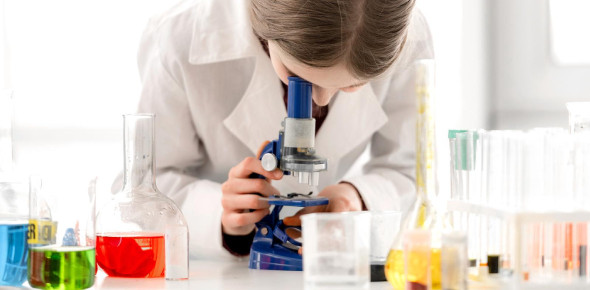# Quiz: Chemistry Regents Exam Practice Test

22 Questions | Attempts: 12135
ShareSettingsPrepare yourself to take this "Chemistry Regents Exam Practice Test" and check how well you score on this quiz. In chemistry, a reagent or analytical reagent is meant by a substance or compound which is added to a system to cause a chemical reaction or test if one occurs. The terms reactant and reagent are usually used interchangeably, but reactant tells a substance consumed during a chemical reaction. Let us test you now!

• 1.
Compared to the charge and mass of a proton, an electron has...
• A.

The same charge and a smaller mass.

• B.

The same charge and the same mass.

• C.

An opposite charge and a smaller mass.

• D.

An opposite charge and the same mass.

• 2.
When alpha particles are used to bombard gold foil, most of the alpha particles pass through undeflected. This result indicates that most of the volume of a gold atom consists of _____.
• A.

Deuterons

• B.

Neutrons

• C.

Protons

• D.

Unoccupied space

• 3.
A proton has approximately the same mass as...
• A.

A neutron.

• B.

An alpha particle.

• C.

A beta particle.

• D.

An electron.

• 4.
What is an example of "Yttrium" in the excited state?
• A.

2-7-19-9-2

• B.

2-8-18-9-2

• C.

2-7-17-8-3

• D.

2-8-18-7-4

• E.

2-8-18-6-6

• 5.
Which symbols represent atoms that are isotopes?
• A.

C-14 and N-14

• B.

O-16 and O-18

• C.

Rn-222 and Ra-222

• D.

I-131 and I-131

• 6.
Which atom contains exactly 15 protons?
• A.

P-32

• B.

S-32

• C.

O-15

• D.

N-15

• 7.
An ion with 5 protons, 6 neutrons, and a charge of 3+ has an atomic number of...
• A.

5

• B.

6

• C.

8

• D.

11

• 8.
What is the mass number of an atom which contains 28 protons, 28 electrons, and 34 neutrons?
• A.

6

• B.

28

• C.

34

• D.

62

• E.

90

• 9.
Which three groups of the Periodic Table contain the most elements classified as metalloids (semimetals)?
• A.

1, 2, and 13

• B.

2, 13, and 14

• C.

14, 15, and 16

• D.

16, 17, and 18

• 10.
Which element has the highest first ionization energy?
• A.

Sodium

• B.

Aluminum

• C.

Calcium

• D.

Phosphorus

• 11.
When a metal atom combines with a nonmetal atom, the nonmetal atom will...
• A.

Lose electrons and decrease in size.

• B.

Lose electrons and increase in size.

• C.

Gain electrons and decrease in size.

• D.

Gain electrons and increase in size.

• 12.
Which element in Group 15 has the strongest metallic character?
• A.

Bi

• B.

As

• C.

P

• D.

N

• 13.
Atoms of elements in a group on the Periodic Table have similar chemical properties. This similarity is most closely related to the atoms'...
• A.

Number of principal energy levels.

• B.

Number of valence electrons.

• C.

Atomic numbers.

• D.

Atomic masses.

• 14.
At STP, which substance is the best conductor of electricity?
• A.

Nitrogen

• B.

Neon

• C.

Sulfur

• D.

Silver

• 15.
Given the unbalanced equation: Al + O2 = Al2O3 When this equation is completely balanced using the smallest whole numbers, what is the sum of the coefficients?
• A.

2

• B.

3

• C.

4

• D.

7

• E.

9

• 16.
What is the empirical formula of the compound whose molecular formula is P4O10? (NOTE: Pv2O = P2O)
• A.

PO

• B.

POv2

• C.

Pv2Ov5

• D.

Pv8Ov20

• 17.
Which is the correct formula for nitrogen (1) oxide? (NOTE: Nv2O = N2O)
• A.

NO

• B.

Nv2O

• C.

NOv2

• D.

Nv2Ov3

• 18.
What is the total number of atoms represented in the formula CuSO4 . 5H2O?
• A.

8

• B.

21

• C.

13

• D.

27

• 19.
What is the gram formula mass of K2CO3?
• A.

106 g

• B.

67 g

• C.

99 g

• D.

138 g

• 20.
What is the percent by mass of oxygen in magnesium oxide, MgO?
• A.

20%

• B.

30%

• C.

40%

• D.

50%

• E.

60%

• 21.
What is the total number of moles of hydrogen gas contained in 9.03 x 1023?
• A.

1.50 moles

• B.

2.00 moles

• C.

6.02 moles

• D.

9.03 moles

• 22.
Which formula represents a molecular substance?
• A.

CaO

• B.

CO

• C.

Al2O3

• D.

Li2O

## Related TopicsBack to top
×

Wait!
Here's an interesting quiz for you.